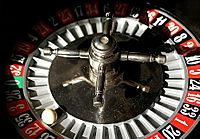# Random facts for kids

Kids Encyclopedia Facts

Random is a term used in mathematics (and less formally) to mean that there is no way to reliably predict an outcome (to know what will happen before it happens) or sense a pattern. Something that is chosen at random is not chosen for any conscious reason, and therefore thought to be purely by chance. An example of a random event is winning a lottery.

A computer can make lists of apparently random numbers. Humans are unable to do this, because the brain works in patterns. If someone is asked to keep saying "heads" or "tails" at random, an astute human observer or properly programmed computer might eventually be able to tell which one the person is likely to say next because the computer notices the patterns.

On a website such as English Wikipedia the user can click on "Random page" to get a random article. The chances of any one page appearing are exactly the same as for any other page.

Sometimes the word "random" is used more loosely. There are websites of random jokes, which just means: a variety of jokes about all sorts of things.

In recent years young people have started to use the word "random" even more loosely to describe anything which is rather strange or has no logic. Sentences such as "the mouldy cheese is escaping" or "I like pie and spam" might be described as "random", although this is not the proper dictionary or mathematical meaning of the word.

## Making random numbersThe ball in a roulette can be used as a source of randomness, because its behavior is very sensitive to the starting conditions.

There are several ways in which a process or system can be seen as random:

1. Randomness coming from the environment (for example, Brownian motion, but also hardware random number generators)
2. Randomness coming from the starting conditions. This aspect is studied by chaos theory. It can be observed in systems that depend very much on differences in the conditions at the start. Examples of such systems are pachinko or dice.
3. Randomness generated by the system itself. This is also called pseudo-randomness, and is the kind used in pseudo-random number generators. There are many algorithms (based on arithmetics or cellular automata) to generate pseudo-random numbers. The behavior of such a system can be predicted, if the random seed and the algorithm are known. These methods are quicker than getting "true" randomness from the environment.

The many applications of randomness have led to many different methods for generating random data. These methods may vary as to how unpredictable or statistically random they are, and how quickly they can generate random numbers.

Before there were computational random number generators, generating large amounts of sufficiently random numbers (important in statistics) required a lot of work. Results would sometimes be collected and distributed as random number tables.

Computers can make what appear to be random numbers. There are two ways to make them.

• There are different algorithms to make random numbers. This makes it possible to model certain aspects of randomness, for example the distribution of the generated numbers. Numbers generated in such a fashion will however always follow a pattern. Given one, or a few of them, a computer can calculate the next random number. Therefore, such numbers are called pseudorandom.
• True random numbers are generated by observing a non-deterministic experiment. The number is then calculated based on the result of the experiment. An example might be to hook up a Geiger counter to a computer, to generate the number.

## In cryptography

Keys for encryption must be random in order to be secure. Keys generated by a faulty random number generator have been cracked.

## Images for kidsRandom Facts for Kids. Kiddle Encyclopedia.